# Extending Rheological Measurements for Concentrated Suspensions Using Squeeze Flow

When shear rheological measurements are made on samples with a high solids fraction, difficulties may be encountered on a rotational rheometer because the sample is likely to fracture even at low - medium shear rates. When the sample fractures at the edge of the geometry gap, a sudden, sharp decrease in shear stress is observed in the data.

Toothpaste is one example of a concentrated suspension that is prone to such effects. Generally, toothpastes contain a polymeric thickener, an abrasive, and a dispersant in an aqueous base, along with preservatives and flavorings. Under rotational shear, these highly packed materials generally exhibit fracture, which can be difficult when evaluating the performance under application-relevant conditions.

In the case of toothpastes, flow characteristics relevant to processing can be hard to determine, and how the finished toothpaste will flow out of the tube and onto the toothbrush is also difficult to predict.

An equilibrium flow curve profile for a standard toothpaste is shown in Figure 1. At 40 s-1, there is a sharp decrease in viscosity that corresponds to the fracture of the toothpaste between the lower and upper geometries.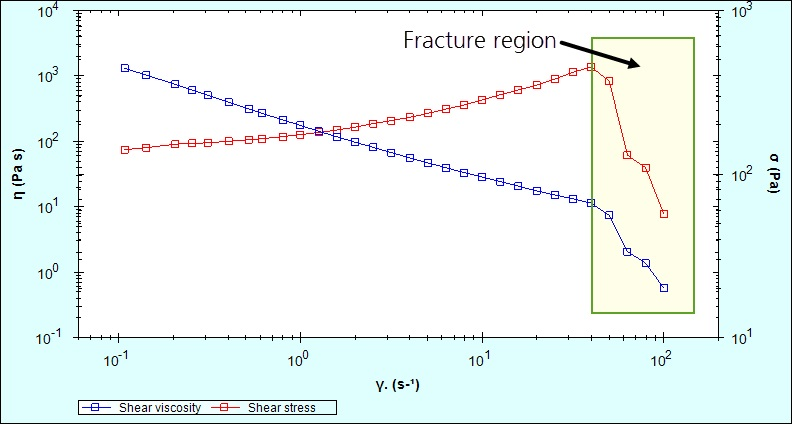Figure 1. Flow curve for a typical toothpaste, showing edge fracture occurring above a shear rate of 40 s-1.

In terms of shear rate, sample fracture can be delayed by using a parallel plate geometry, which allows the application of a smaller gap size but it cannot be removed completely. In the case of highly filled materials containing large particles, the use of a narrow gap may prove to be detrimental because a large enough gap has to be used to prevent the particles jamming under shear .

Squeeze flow is another technique used for measuring the shear flow properties of such systems. In this technique, a sample is loaded between parallel plates and the normal force created by the sample is measured as the gap closes at a constant speed for example.

Laun et al (Laun, Rady, & Hassager, 1999) has developed a method, which accounts for partial wall slip, to transform gap and normal force data into shear stress and shear rate. This makes it possible to calculate shear viscosity as a function of shear rate.

The maximum normal force capability of the rheometer limits the maximum shear rate available at a set gapping speed. However, the maximum shear rate can often surpass the shear rate that can be achieved using rotational rheometry, where the sample shows edge fracture.

The method is such that a defined amount of sample is loaded onto the center of the lower geometry plate, followed by lowering the upper plate at a constant speed to a defined end gap (Figure 2). The upward force produced by the sample resisting the geometry’s downward movement and the corresponding gap is calculated as a function of time.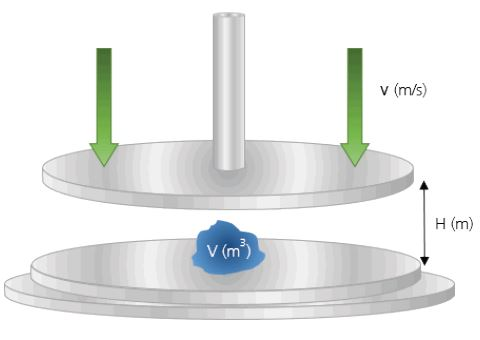Figure 2. Schematic of axial measurements performed using the Kinexus rheometer.

Data is captured as normal force vs. gap, and using Equations  and , is converted to shear stress and shear rate, respectively. The data can also be applied with a power law correction, though it is not described here.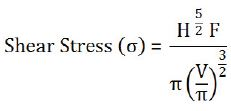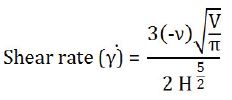## Experiment

• The toothpaste’s squeeze flow behavior was assessed at gapping speeds of 2 mm/s and 10 mm/s
• Measurements were carried out on 1 g aliquots of toothpaste, using a Kinexus rotational rheometer equipped with a 60 mm parallel plate measuring system and a Peltier plate cartridge, utilizing a squeeze flow sequence in the rSpace for Kinexus software
• Comparison rotational flow curve data was produced using a 40 mm roughened parallel plate with a 1 mm gap and using a standard preconfigured rSpace sequence
• All measurements were carried out at a temperature of 25 °C
• Using a toothpaste density of 1.3 g/cm3, sample mass was converted to volume

## Results and Discussion

Figure 3 shows a gap and normal force profile for toothpaste, with a gapping speed of 2 mm/s. The blue line representing gap shows how the upper geometry plate approaches the sample. The plate forms a compressed cylinder of increasing diameter as it comes into contact with the sample, and the red line, indicating normal force, begins to increase.

When the upper geometry approaches the defined end gap, the compressive force becomes constant as the squeezing stops.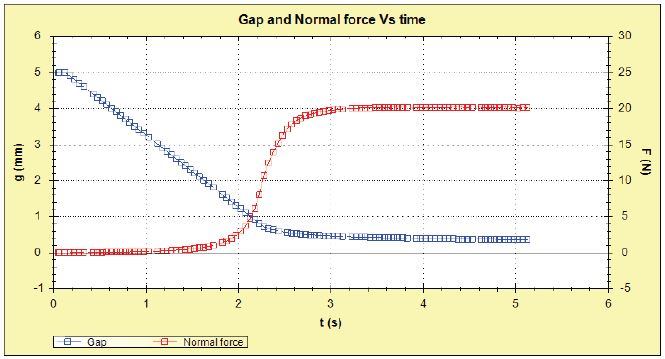Figure 3. Gap and normal force profile for toothpaste, using a gapping speed of 2 mm/s.

Using equations  and , normal force and gap data are converted into shear stress and shear rate respectively and the shear viscosity determined by dividing the resulting shear stress by the corresponding shear rate.

Figure 4 shows the resulting flow curve created from the squeeze flow data using a gapping speed of 2 mm/s. Three clear regions are seen in this chart in terms of sample flow behavior;

• Up to approximately 7 s-1, the sample is just beginning to flow as compressive forces begin to increase
• From 7 s-1, the viscosity profile reveals a gradient change as the sample exhibits flow
• An additional gradient change takes place above 150 s-1 as the compressive forces reach a maximum and sample flow ceases

Only the data representative of constant sample flow is utilized from the measurement.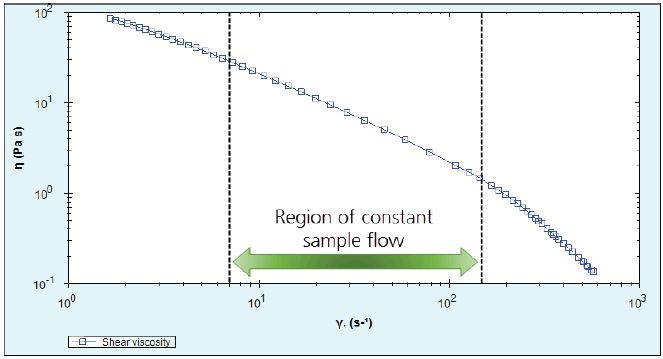Figure 4. Viscosity flow curve calculated from squeeze flow data obtained at a gapping speed of 2 mm/s.

For a fresh 1 g aliquot of toothpaste, the squeeze flow test was repeated but this time a gapping speed of 10 mm/s was used. Figure 5 shows a comparison of 2 and 10 mm/s data, along with the equilibrium flow data acquired using conventional rotational rheometry.

It is observed that the squeeze flow data corresponds very well with the rotational data, extending the shear rate from a maximum of 20 s-1 for rotational measurements to 700 s-1 for squeeze flow measurements. Obviously, different samples may be more or less appropriate for the squeeze flow method than that illustrated here, and therefore, trial measurements are proposed for any new analysis.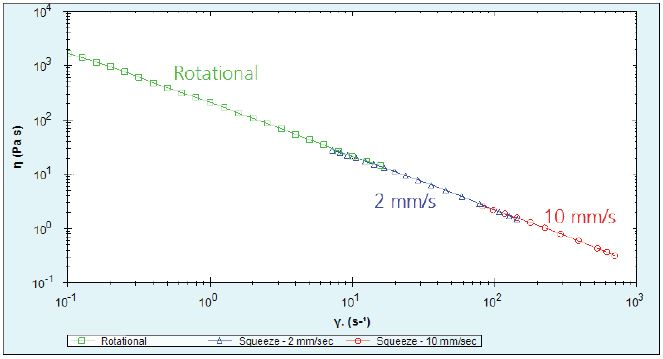Figure 5. Rotational and squeeze flow data, presented as viscosity versus shear rate.

## Conclusion

A Kinexus rotational rheometer equipped with sophisticated axial test capabilities can help to extend the measurable shear rate range of concentrated suspensions, which are inclined to fracture, by using the squeeze flow method. The measured viscosities for toothpaste achieved by squeeze flow measurements provided similar data to conventional rotational rheometry, and also extended the shear rate range by almost two orders of magnitude.

Notes and References

Laun, H. M., Rady, M., & Hassager, O. (1999). Analytical solutions for squeeze flow with partial wall slip. Journal of Non-Newtonian Fluid Mechanics (81), 1-15.

Footnote

 The size of the gap should be 10x the size of the maximum particle so that there is enough free space between the particles for them to move freely. With increasing shear rate and a narrow gap, large particles tend to jam together, falsifying flow behaviorThis information has been sourced, reviewed and adapted from materials provided by Malvern Panalytical.

## Citations

• APA

Malvern Panalytical. (2019, September 03). Extending Rheological Measurements for Concentrated Suspensions Using Squeeze Flow. AZoM. Retrieved on January 22, 2020 from https://www.azom.com/article.aspx?ArticleID=13437.

• MLA

Malvern Panalytical. "Extending Rheological Measurements for Concentrated Suspensions Using Squeeze Flow". AZoM. 22 January 2020. <https://www.azom.com/article.aspx?ArticleID=13437>.

• Chicago

Malvern Panalytical. "Extending Rheological Measurements for Concentrated Suspensions Using Squeeze Flow". AZoM. https://www.azom.com/article.aspx?ArticleID=13437. (accessed January 22, 2020).

• Harvard

Malvern Panalytical. 2019. Extending Rheological Measurements for Concentrated Suspensions Using Squeeze Flow. AZoM, viewed 22 January 2020, https://www.azom.com/article.aspx?ArticleID=13437.